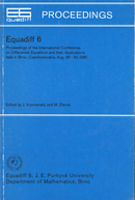## Equadiff 6 Proceedings of the International Conference on Differential Equations and Their Applications held in Brno, Czechoslovakia, Aug. 26 - 30, 1985Editors: Vosmanský, Jaromír; Zlámal, Miloš
Venue: Brno, 1985

Publisher: J. E. Purkyně University, Department of Mathematics, Brno, 1986

MR0881609
MSC: 00B25, 34-06, 35-06, 65-06

 III-IV Preface.
 V-VII Contents.
 [IX]-XVI List of papers presented at the conference.
 XVII-XX List of participants.

Plenary lectures
 -16 Mathematical and numerical study of nonlinear problems in fluid mechanics.  Feistauer, Miloslav
 17-22 Free boundary problems in fluid dynamics.  Friedman, Avner
 23-34 Method of Rothe in evolution equations.  Kačur, Jozef
 35-48 Boundary value problems in weighted spaces.  Kufner, Alois
 49-58 Critical point theory and nonlinear differential equations.  Mawhin, Jean
 59-70 Ordinary linear differential equations - a survey of the global theory.  Neuman, František
 71-84 Numerical and theoretical treating of evolution problems by the method of discretization in time.  Rektorys, Karel
 85-94 Algorithms for the inclusion of solutions of ordinary initial value problems.  Stetter, Hans J.
 95-106 Recent developments in the theory of function spaces.  Triebel, Hans

Section A: Ordinary differential equations
 -113 On properties of oscillatory solutions of non-linear differential equations of the n-th order.  Bartušek, Miroslav
 -121 Uniqueness without continuous dependence.  Burton, Theodore Allen; Dwiggins, D. P.
 -128 Connections in scalar reaction diffusion equations with Neumann boundary conditions.  Fiedler, Bernold; Brunovský, Pavol
 129-132 On a certain boundary value problem of the third order.  Greguš, Michal
 -139 On nonparasite solutions.  Krbec, Pavel
 -148 Uniform zeros for beaded strings.  Kreith, Kurt
 -154 Perron integral, Perron product integral and ordinary differential equations.  Kurzweil, Jaroslav; Jarník, Jiří
 -160 On the zeros of some special functions: differential equations and Nicholson-type formulas.  Muldoon, Martin E.
 -170 Surjectivity and boundary value problems.  Šeda, Valter
 -179 Some problems concerning the equivalences of two systems of differential equations.  Švec, Marko
 -186 Linear perturbations of general disconjugate equations.  Trench, William F.
 -190 On optimal control of systems with interface side conditions.  Tvrdý, Milan

Section B: Partial differential equations
 -196 A description of blow-up for the solid fuel ignition model.  Bebernes, Jerrold W.
 -202 Spectral analysis of non-self-adjoint elliptic operators.  Brilla, Jozef
 -208 On the mountain pass lemma.  Kung-Ching Chang
 -214 On uniqueness and stability of steady-state carrier distributions in semiconductors.  Gajewski, Herbert
 -219 Partial regularity of minimizers.  Giaquinta, Mariano
 -226 Periodic solutions of partial differential equations with hysteresis.  Krejčí, Pavel
 227-234 Stability and bifurcation problems for reaction-diffusion systems with unilateral conditions.  Kučera, Milan
 -242 Boundary integral equations of elasticity in domains with piecewise smooth boundaries.  Maz'ya, Vladimir G.
 -246 Higher regularity of weak solutions of strongly nonlinear elliptic equations.  Simader, Christian G.
 -250 Some regularity results for quasilinear parabolic systems.  Stará, Jana; John, Oldřich
 251-258 Classical boundary value problems for Monge-Ampère type equations.  Trudinger, Neil S.
 -264 Qualitative properties of the solutions to the Navier-Stokes equations for compressible fluids.  Valli, Alberto

Section C: Numerical Methods
 -274 On Gel'fand's method of chasing for solving multipoint boundary value problems.  Agarwal, Ravi P.
 -284 Stability and error estimates valid for infinite time, for strongly monotone and infinitely stiff evolution equations.  Axelsson, Owe
 -289 Recent results in the approximation of free boundaries.  Brezzi, Franco
 -294 Finite element solution of a nonlinear diffusion problem with a moving boundary.  Čermák, Libor; Zlámal, Miloš
 -302 Analysis of Thacker's method for solving the linearized shallow water equations.  Descloux, Jean; Ferro, Robert
 -308 The convergence of a new method for calculating lower bounds to eigenvalues.  Goerisch, Friedrich; Albrecht, Julius
 -314 Bifurcation analysis of stimulated Brillouin scattering.  Janovský, Vladimír; Marek, Ivo; Neuberg, Jiří
 -320 Superconvergence results for linear triangular elements.  Křížek, Michal
 -325 Mixed finite element in 3D in \$H(\operatorname{div})\$ and \$H(\operatorname{curl})\$.  Nédélec, Jean-Claude
 -332 Free boundary problems for Stoke's flows and finite element methods.  Nitsche, Joachim A.
 -338 Enclosing methods for perturbed boundary value problems in nonlinear difference equations.  Schmidt, Jochen W.
 -344 Error estimates for finite element methods for semilinear parabolic problems with nonsmooth data.  Thomée, Vidar
 -352 Singularities in two- and three-dimensional elliptic problems and finite element methods for their treatment.  Whiteman, John Robert
 353-358 Some new convergence results in finite element theories for elliptic problems.  Ženíšek, Alexander

Section D: Applications
 -366 Mathematical solution of direct and inverse problem for transonic cascade flows.  Bolek, P.; Fořt, Jaroslav; Kozel, Karel; Polášek, Jan
 -372 Einige Anwendungen der mehrdimensionalen approximationstheorie zur Lösungseinschließung bei Randwertaufgaben.  Collatz, Lothar
 -378 A posteriori estimations of approximate solutions for some types of boundary value problems.  Kodnár, Rudolf
 -386 Nonlinear dynamics systems - bifurcations, continuation methods, periodic solutions.  Kubíček, Milan; Holodniok, Martin
 -392 The ROTHE method for nonlinear hyperbolic problems.  Martensen, Erich
 -398 Some solved and unsolved canonical problems of diffraction theory.  Meister, E.
 -408 Entropy compactification of the transonic flow.  Nečas, Jindřich
 -414 The global existence of weak solutions of the mollified system of equations of motion of viscous compressible fluid.  Neustupa, Jiří
 -420 Bifurcations near a double eigenvalue of the rectangular plate problem with a domain parametr.  Sadovský, Zoltán
 -424 Delay makes problems in population modelling.  Smítalová, Kristína

Supplement
 -430 Analytical and computational problems in higher special functions.  Arscott, Felix M.
 -434 On oscillation of solutions of linear ordinary differential equations.  Chanturia, Teimuraz A.
 -444 Deterministic and stochastic vector differential equations applied in technical systems theory.  Fazekas, F.
 -450 Shape optimization in contact problems.  Haslinger, Jaroslav
 -458 Zur analytischen Lösung alter und neuer geometrischer Optimierungsprobleme.  Klötzler, Rolf
 -464 On the smoothness of the solutions to the elliptic systems.  Koshelev, Alexander Ivanovich
 465-468 On the asymptotic behavior of solutions of nonlinear ordinary differential equations.  Kusano, Takaŝi